NEET  >  Test: Chemical Equation

# Test: Chemical Equation

Test Description

## 20 Questions MCQ Test Chemistry Class 11 | Test: Chemical Equation

Test: Chemical Equation for NEET 2023 is part of Chemistry Class 11 preparation. The Test: Chemical Equation questions and answers have been prepared according to the NEET exam syllabus.The Test: Chemical Equation MCQs are made for NEET 2023 Exam. Find important definitions, questions, notes, meanings, examples, exercises, MCQs and online tests for Test: Chemical Equation below.
Solutions of Test: Chemical Equation questions in English are available as part of our Chemistry Class 11 for NEET & Test: Chemical Equation solutions in Hindi for Chemistry Class 11 course. Download more important topics, notes, lectures and mock test series for NEET Exam by signing up for free. Attempt Test: Chemical Equation | 20 questions in 30 minutes | Mock test for NEET preparation | Free important questions MCQ to study Chemistry Class 11 for NEET Exam | Download free PDF with solutions
 1 Crore+ students have signed up on EduRev. Have you?
Test: Chemical Equation - Question 1

### Two flasks A and B of equal volume containing 1 mole and 2 mole of O3 respectively, are heated to the same temperature. When the reaction 2O3 →  3O2 practically stops, then both the flasks shall have

Detailed Solution for Test: Chemical Equation - Question 1

For Reaction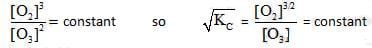= will be same for both the containers

Test: Chemical Equation - Question 2

### A 10L container at 300K contains CO2 gas at pressure of O.2 atm and an excess solid CaO (neglect the volume of solid CaO). The volume of container is now decreased by moving the movable piston fitted in the container. What will be the maximum volume of container when pressure of CO2 attains its maximum value given that                                                CaCO3 (s)   → CaO(s) + CO2(g)             Kph = 0.800 atm

Detailed Solution for Test: Chemical Equation - Question 2

Kp = 0.800 atm =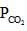= maximum pressure of CO2 in the container to calculate maximum volume of container the= 0.8 atm and none of= 0.8 should get converted into CaCO3 (s)
so V (0.800 atm) = (10 L) (o.2 atm)
so v = 2.5 L

Test: Chemical Equation - Question 3

### An equilibrium mixture at 700 K of 0.50 M N2, 3.00 M H2 and 2.00 M NH3 is present in a container. Now if this equilibrium is disturbed by adding N2 so that its concentration becomes 1.50 M just after addition then which of the following graphs represents the above situation more appropriately –

Detailed Solution for Test: Chemical Equation - Question 3

Let reaction be

N2+3H→ 2NH3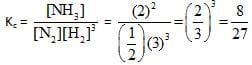Now after distrubing equilibrium then N2 concentration will be > 0.5 M and there will be some time taken for attainment of equilibrium hence graphs (B) and (C) can be discarded

from calculations it can be proved that the amount by which N2 should reaction should be less than 0.2 . Hence the result.

Test: Chemical Equation - Question 4

At 1400 K, Kc= 2.5x10 +3 for the reaction CH4(g) + 2H2S(g) CS2(g) + 4H2(g) . A 10.0L reaction vessel at 1400 K contains 2.00 mole of CH4, 3.0 mol of CS2, 3.0 mole of H2 and 4.0 mole of H2S. Then

Detailed Solution for Test: Chemical Equation - Question 4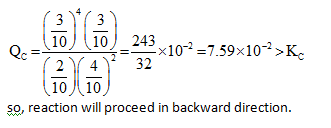Test: Chemical Equation - Question 5

The graph which will be representing all the equilibrium concentrations for the reaction

N2O4 → 2NO2 (g) will be :

(the concentrations of N2O4 (g) and of NO (g) for which the following eaction will be at equilibrium will lie

Detailed Solution for Test: Chemical Equation - Question 5

All the equilibrium will statisfy the equation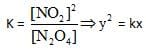the required parabolic graph

Test: Chemical Equation - Question 6

In a basic aqueous solution chloromethane undergoes a substitution reaction in which Cl is replaced by OH as.

CH3Cl (aq) + OH- → CH3OH(aq) + Cl(aq)

The equilibrium constant of above reaction C, =1 x 10'6. If a solution is prepared by mixing equal volumes of 0.1 M CH3CI and 0.2 M NaOH (100% dissociated) then [OH-] concentration at equilibrium in mixture will be:

Detailed Solution for Test: Chemical Equation - Question 6

CH3Cl (aq) + OH- → CH3OH(aq) + Cl-(aq)

As equilibrium constant is very large so reaction will be almost 100% complete.

Test: Chemical Equation - Question 7

For the chemical equilibrium,
CaCO3 (S) → CaO(s) + CO2 (g)

ΔHf° can be determined from which one of the following plots ?

Detailed Solution for Test: Chemical Equation - Question 7

CaCO3 (S) → CaO(s) + CO2 (g)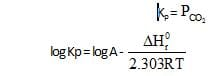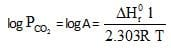Graph (a) represents (i) and its slope will be used to determine the heat of the reaction.

Test: Chemical Equation - Question 8

At a certain temperature the following equilibrium is established, CO(g) + N02(g) →  CO(g) + NO(g) One mole of each of the four gas is mixed in one litre container and the reaction is allowed to reach equilibrium state. When excess of baryta water (Ba(OH)2) is added to the equilibrium mixture, the weight of white ppt (BaCO3) obtained is 236.4 gm. The equilibrium constant Cc of the reaction is (Ba = 137)

Detailed Solution for Test: Chemical Equation - Question 8

CO(g) + N02(g) →  CO(g) + NO(g)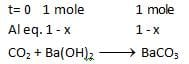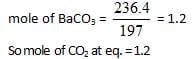or     1+x = 1.2
x= 0.2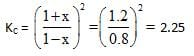Test: Chemical Equation - Question 9

At temperature T, the compound AB2 (g) dissociates according to the reaction, 2AB2 (g)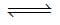2 AB(g) + B2(g).With a degree of dissociation x, which is small compared with unity. Deduce the expression for x in terms of the equilibrium constant, Kp and the total pressure, P.

Detailed Solution for Test: Chemical Equation - Question 9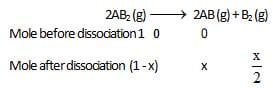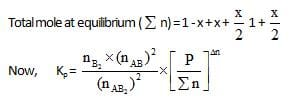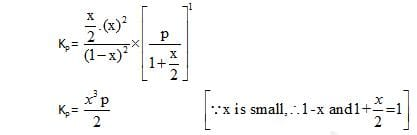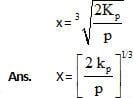Test: Chemical Equation - Question 10

Equilibrium constant for the given reaction is C = 1020 at temperature 300 K

A(s) +2B(aq)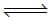2C (s) + D(aq.)K = 1020

The equilibrium conc. of B starting with mixture of 1 mole of A and 1/3   mote/titre of B at 300 K is

Detailed Solution for Test: Chemical Equation - Question 10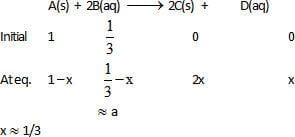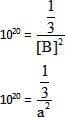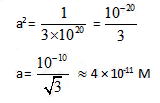Test: Chemical Equation - Question 11

10lt box contain O3 and O2 at equilibrium at 2000 K. The ΔG0 = –534.52  kJ at 8 atm equilibrium

pressure.The following equilibrium is present in the container

2o3(g)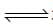3O2 (g). the partial pressure of O3 will be ( In 10 = 2.3, R = 8.3  J mol-1 K-1)

Detailed Solution for Test: Chemical Equation - Question 11

ΔG0 = -Rt In K = -2.3 x 2000 x 8.3 log K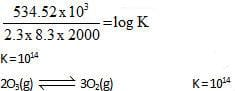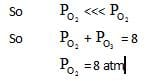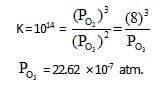Test: Chemical Equation - Question 12

Solid ammonium carbamate dissociates to give ammonia and carbon dioxid as follows:

NH2COONH4(s)2NH5(g) + CO2(g)

At equilibrium, ammonia is added such that partial pressures of NH5 now equals the original total pressure. Calculate the ratio of the total pressures now to the original total pressure.

Detailed Solution for Test: Chemical Equation - Question 12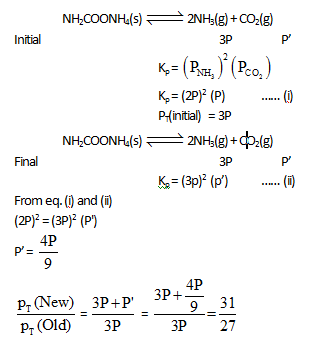Test: Chemical Equation - Question 13

The reactions,   PCl5 (g)PCl5(g) + Cl2(g) and Cl2(g)CO2(g) + Cl2 (g) are simultaneously in equilibrium in an equilibrium box at constant volume. Afew  moles of   CO(g) are later introduced into the vessel. After some time, the new  equilibrium concentration of

Detailed Solution for Test: Chemical Equation - Question 13

If CO is added 2nd equilibrium will proceede in the backward dkection and concentration of Cl2 will decrease. This Cl2 will be furtherformed by the decomposition of PCI5

Test: Chemical Equation - Question 14

In the Haber process for the industrial manufacture of ammonia involving the  reaction,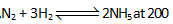atm pressure in the presence of a catalyst a temperature of about 500°C is used. This is considered as optimum temperature for the process because

Detailed Solution for Test: Chemical Equation - Question 14

Formation of ammonia is an exothermix process therefore it is favorable at lower temperature. But at lower temperature rate of the reaction becomes slow.

Test: Chemical Equation - Question 15

For the equilibrium of the reaction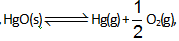,kP for the reaction at total pressure of P is:

Detailed Solution for Test: Chemical Equation - Question 15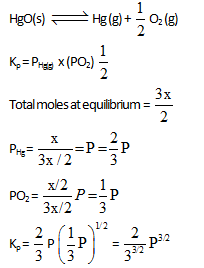Test: Chemical Equation - Question 16

What is the minimum mass of CaC05 (s), below which it decomposes completely, required to establish equilibrium in a 6.50 litre container for the reaction : [ KC = mole/litre]

CaCO3 (s)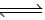CaO(s) + CO2(g)

Detailed Solution for Test: Chemical Equation - Question 16

Kc = [Cl2] = 0.05 mole/itre
so moles of CO2= 6.50 x 0.05 moles = 0.3250 moles

CaCl3CaO + Cl2

1 mole of CO2 =1 mole of CaCO3

0.3250 moles of CO2 = 0.3250 moles of CaCO3= 0.3250 x 100 gm of CaCO3 = 32.5 gm of CaCO

Test: Chemical Equation - Question 17

The value of kp for the reaction at 27°C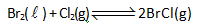is '1 atom'. At equilibrium in a closed container partial pressure of BrCI gas is 0.1 atm and at this temperature the vapour pressure of Br2(l) is also 0.1 atm. Then what will be minimum moles of Br2(l) to be added to 1 mole of C12 , initially, to get above equilibrium situation :

Detailed Solution for Test: Chemical Equation - Question 17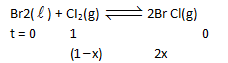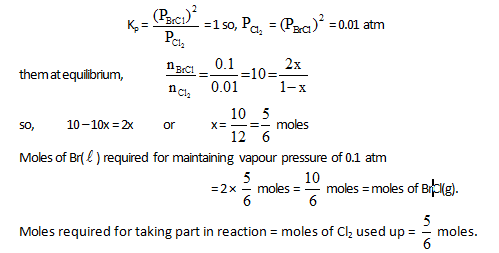Test: Chemical Equation - Question 18

5 mol PCI3(g) and one mole N2 gas is placed in a closed vessel. At equilibrium PCI5(g) decomposes 20% and total pressure in to the container is found to be 1 atm. The kP for equilibrium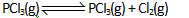Detailed Solution for Test: Chemical Equation - Question 18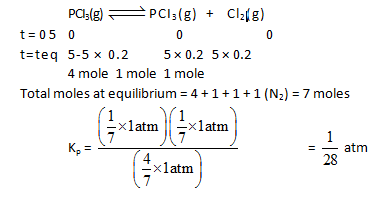Test: Chemical Equation - Question 19

Degree of association can be defined as the number of moles of a particular substance associated per mole of the substance taken.

For example : If out of 10 mole of N2 , 3 mol of N2 combine with H2 to form NHL. then degree of association of N2 = 0.3.Consider the equilibrium situation :  N2(g) + 3H2(g)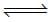2NH3(g)

Initially N2 & H2 were mixed in 1 : 3 molar ratio and after long time the mean molar mass of the mixture was  found to be 34/3g. The degree of association of N2 is

Detailed Solution for Test: Chemical Equation - Question 19

The correct answer is Option A
N2​(g) + 2H2​(g) ⇌ 2NH3​(g)
t=0        1             3              0
t=teq​  1 - x       3 - 3x         2x
Total moles at equilibrium = 4 − 2x
Mean molar mass  =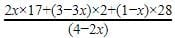Value of mean molar mass is given. Find ′x′.

Test: Chemical Equation - Question 20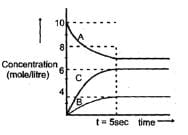Attainment of the equilibrium A(g)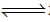2C(g) +B(g) gave the gave the followeing graph. Find the correct option. (% dissociation = fraction dissociated x 100)

Detailed Solution for Test: Chemical Equation - Question 20

The correct answer is Option D.

Kc = ([C]2 * [B]) / [A]
= (62 * 4) / 8
= 18 M2

And, % dissociation of A = ([10 - 8] / 10)*100
=  20 %

## Chemistry Class 11

204 videos|331 docs|229 tests
 Use Code STAYHOME200 and get INR 200 additional OFF Use Coupon Code
Information about Test: Chemical Equation Page
In this test you can find the Exam questions for Test: Chemical Equation solved & explained in the simplest way possible. Besides giving Questions and answers for Test: Chemical Equation, EduRev gives you an ample number of Online tests for practice

## Chemistry Class 11

204 videos|331 docs|229 tests

### How to Prepare for NEET

Read our guide to prepare for NEET which is created by Toppers & the best Teachers